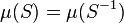# Haar measure

## Definition

Let$G$ be a locally compact group.

### Left Haar measure

A left Haar measure is a left-translation-invariant countably additive regular nontrivial measure$\mu$ on the Borel subsets of$G$. The conditions are explained below:

Condition name Explanation
Borel subset A subset in the$\sigma$-algebra generated by open subsets of$G$
Left-translation-invariant measure If$S \subseteq G$ is measurable, and$g \in G$, then$gS$ is measurable, and$\mu(gS) = \mu(S)$, where$gS = \{ gs \mid g \in G \}$
Countably additive (part of the usual definition of measure) If$S_1, S_2, \dots, S_n, \dots$ are all measurable sets that are pairwise disjoint, and their union is$S$, then$\mu(S)$ is the sum of the values$\mu(S_i)$.
Regular Borel measure$\mu(K)$ is finite for any compact subset$K$. Also, for every Borel subset$S$,$\mu(S) = \inf \{\mu(U), S \subseteq U, U \operatorname{open} \}$ and$\mu(S) = \sup \{ \mu(UK), K \subseteq S, K \operatorname{compact} \}$
Nontrivial measure$\mu(U) > 0$ for any nonempty open subset$U$ of$G$

The left Haar measure for a locally compact group is unique up to scalar multiples, i.e., the quotient of any two left Haar measures is a scalar.

For a compact group, there is a unique choice of normalized Haar measure, i.e., a unique left Haar measure where the total measure of the group is$1$.

### Right Haar measure

A right Haar measure is a left-translation-invariant countably additive regular measure$\mu$ on the Borel subsets of$G$.

The right Haar measure for a locally compact group is unique up to scalar multiples, i.e., the quotient of any two left Haar measures is a scalar.

### Relationship between left and right Haar measure

Any left Haar measure on a group can be used to canonically define a right Haar measure: for a left Haar measure$\mu_l$, define a correpsonding right Haar measure as:$\mu_r(S) := \mu_l(S^{-1})$

where$S^{-1} = \{ s^{-1} \mid s \in S \}$

This definition makes sense because$S$ is Borel if and only if$S^{-1}$ is. The left Haar measure conditions on$\mu_l$ give rise to the right Haar measures on$\mu_r$.

## Particular cases

### Compact groups

For a compact group, the following additional features are true:

• The left Haar measures coincide with the right Haar measures. In particular, we just talk of a Haar measure without talking of left or right. This Haar measure thus satisfies the additional condition that$\mu(S) = \mu(S^{-1})$ for any measurable subset$S$.
• Further, since the total measure of the group is finite, there is a natural choice of normalized Haar measure where the total measure of the group is$1$.

### Bi-invariant Haar measures?

A bi-invariant Haar measure is a measure that is both a left and a right Haar measure. Not all locally compact groups have bi-invariant Haar measures, but compact groups, locally compact abelian groups, and some other examples of locally compact groups have bi-invariant Haar measures. If a group has a bi-invariant Haar measure, then every left Haar measure is a right Haar measure, and hence a bi-invariant Haar measure.